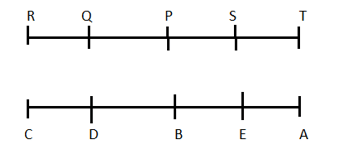# Reasoning Quiz IBPS PO & IBPS CLERK PRE

Reasoning Quiz to improve your  Reasoning  for SBI Po & SBI clerk exam Reasoning, IBPS PO Reasoning , IBPS Clerk Reasoning , IBPS RRB Reasoning, LIC AAO , LIC ADO,  and other competitive exams.

Directions (Q1-Q5): Study the following information to answer the given questions:

Ten people are sitting in two parallel rows containing five people each, in such a way that there is an equal distance between adjacent persons. In row 1, P, Q, R, S and T are seated and all of them are facing south. In row 2, A, B, C, D and E are seated and all of them are facing north. Therefore, in the given seating arrangement, each member seated in a row faces another member of the other row.

D sits third to the left of A. P faces immediate neighbor of D. R sits second to the right of P. Only one person sits between Q and S. B and E are immediate neighbors. E does not face P and Q.

Q1.How many persons are seated between Q and T?

(a) None

(b) One

(c) Two

(d) Three

(e) Cannot be determined

Q (1 – 5):Q1. Ans.(c)

P and S

Q2.Four of the following five are alike in a certain way and thus form a group. Which is the one that does not belong to that group?

(a)R

(b) S

(c)C

(d) T

(e)A

Q (1 – 5):Q2. Ans.(b)

All other are sitting at the ends.

Q3. Who among the following are sitting exactly in the middle of the rows?

(a)P,E

(b) S, D

(c) S, A

(d)A, R

(e) P, B

Q (1 – 5):Q3. Ans.(e)

Q4. Which of the following is true regarding B?

(a) A and C are immediate neighbors of B.

(b) B sits at one of the extreme ends of the line.

(c) Q faces B.

(d) T is an immediate neighbor of the person facing B.

(e) D sits on the immediate left of B.

Q (1 – 5):Q4. Ans.(e)

Q5. Four of the following five are alike in a certain way and thus form a group. Which is the one that does not belong to that group?

(a) T— E

(b) Q — C

(c) S — B

(d)R—A

(e) P — D

Q (1 – 5):Q5. Ans.(d)

In all other, the second is the neighbor of the one facing the first.

Q6. In these questions, relationship between different elements is show in the statements. The statements are followed by conclusions. Study the conclusions based on the given statements and select the appropriate answer:A. If only conclusion I follows.
If only conclusion II follows.
If either conclusion I or II follows.
If neither conclusion I nor II follows.
EIf both conclusions I and II follow.
Q6. Ans(E)Q.7 In these questions, relationship between different elements is show in the statements. The statements are followed by conclusions. Study the conclusions based on the given statements and select the appropriate answer:A. If only conclusion I follows.
B. If only conclusion II follows.
C. If either conclusion I or II follows.
D. If neither conclusion I nor II follows.
E. If both conclusions I and II follow.
Q7. Ans(B)Q.8 In these questions, relationship between different elements is show in the statements. The statements are followed by conclusions. Study the conclusions based on the given statements and select the appropriate answer:A. If only conclusion I follows.
B. If only conclusion II follows.
C. If either conclusion I or II follows.
D. If neither conclusion I nor II follows.
E. If both conclusions I and II follow.
Q8. Ans(C)Q9. In each of the questions below are given some statements followed by some conclusions. You have to take the given statements to be true even if they seem to be at variance with commonly known facts. Read all the conclusions and then decide which of the given conclusions logically follows from the given statements disregarding commonly known facts.
Statements:
Some School are College.
Only a few College are University.
No University is Office.
Conclusions:
I. All College being University is a possibility.
II. Some College are not Office.
A. If only conclusion I follows.
B. If only conclusion II follows.
C. If either conclusion I or II follows.
D. If neither conclusion I nor II follows.
E. If both conclusions I and II follow.
Q9. Ans(B)Q10.  In each of the questions below are given some statements followed by some conclusions. You have to take the given statements to be true even if they seem to be at variance with commonly known facts. Read all the conclusions and then decide which of the given conclusions logically follows from the given statements disregarding commonly known facts.
Statements:
Some Tree are Leaf.
All Leaf are Green.
Some Plant are not Green.
Conclusions:
I. Some Leaf are not Plant.
II. Some Leaf can never be Tree.
A. If only conclusion I follows.
B. If only conclusion II follows.
C. If either conclusion I or II follows.
D. If neither conclusion I nor II follows.
E. If both conclusions I and II follow.
Q10. Ans(D)### You Can Read This Also:

Best E-books for LIC AAO 2019 : Get PDF here

ambitiousbaba.com need your support to Grow

I challenge you will get Best Content in Our PDFs with Detail solutions and Latest Pattern

Memory Based Puzzle E-book | 2016-19 Exams Covered

Get PDF here

Caselet Data Interpretation 200 Questions

Get PDF here
Puzzle & Seating Arrangement E-Book for BANK PO MAINS (Vol-1)

Get PDF here

### ARITHMETIC DATA INTERPRETATION 2019 E-book

Get PDF here
The Banking Awareness 500 MCQs E-book| Bilingual (Hindi + English)

Get PDF here

High Level DATA INTERPRETATION Practice E-BOOK

Get PDF her